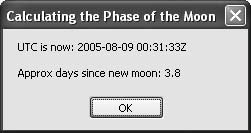# Recipe 7.19. Calculating the Phase of the Moon

#### Problem

You want to calculate the phase of the moon for a given date.

#### Solution

Sample code folder: Chapter 07\MoonPhase

Use Visual Basic 2005's Date and Math functions to apply a linear-fit equation to calculate the phase of the moon, accurate to within half an hour.

#### Discussion

The linear curve fit equation presented here was researched and created only recently, using data from the Internet that provided the date and time of all new moons over a period of several centuries. The results are surprisingly accurate, and the equation is easy to use, especially with the helpful math and date functions available in Visual Basic.

Here's the MoonPhase() function resulting from the research:

` Public Function MoonPhase(ByVal dateUtc As Date) As Double    ' ----- Determine the phase of the moon for any date.    Dim days As Double = dateUtc.Subtract(#1/1/1600#).TotalDays    Dim cycles As Double = days * 0.03386319 - 12.5    Return   Math.IEEERemainder(cycles, 1.0) * 29.53059 End Function `

The date for determining the moon's phase is passed to this function as dateUtc, and it should be an exact date and time value expressed in Coordinated Universal Time. A TimeSpan is calculated by subtracting from the date the literal date constant for midnight, January 1, 1600. The TotalDays property of the resulting TimeSpan provides the total elapsed days, complete with a decimal result for the fraction of the day. The decimal number of days is stored in the Double variable named days.

The heart of this algorithm is in the second line of the function. The number of elapsed days since the start of 1600 is multiplied by 0.03386319, and an offset of 12.5 days is subtracted from the result. This linear equation provides an approximate number of full moons since 1600. The fractional part, which cycles through values from 0 to 1 between successive new moons, is the part that's interesting. Rather than simply extracting the fractional part of cycles, the Math.IEEERemainder() function returns a value ranging from-0.5 to +0.5, and this value is multiplied by the number of mean days between full moons to get the number of days, plus or minus, to the closest full moon.

The following code reports the closest new moon using the MoonPhase() function:

` Dim phaseDay As Double Dim result As String ' ----- Determine the phase of the moon. phaseDay = MoonPhase(Now.ToUniversalTime) ' ----- Show the nearest new moon. result = "UTC is now: " & _    Now.ToUniversalTime.ToString("u") & vbNewLine & vbNewLine If (phaseDay < 0) Then    result &= "Approx days until new moon: " & _       (-phaseDay).ToString("F1") Else    result &= "Approx days since new moon: " & _       phaseDay.ToString("F1") End If MsgBox(result) `

This code converts the current local time to UTC using the ToUniversalTime() method before sending that time to the moon-phase calculator. Figure 7-20 shows the sample code in use.

##### Figure 7-20. The moon is waxing and it's about &#188; lit by the sunAfter running a curve fit program to compute the equation used earlier, a second program was written to find the maximum absolute error in time for all new moons in the range of years from 1600 to 2500. Surprisingly, the maximum drift of the time of new moon was less than half an hour. This equation, even though it's a simple one, is good enough to allow you to predict when you'll be able to fish, plant, and dance by the light of the moon.Visual Basic 2005 Cookbook: Solutions for VB 2005 Programmers (Cookbooks (OReilly))
ISBN: 0596101775
EAN: 2147483647
Year: 2006
Pages: 400